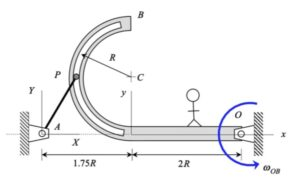# Homework H.3.DDiscussion• Shown above left is the motion of the mechanism as seen by a fixed observer. Note that the stationary observer sees P moving on a circular path centered at point A.
• And, shown above right is the motion of P as seen by an observer attached to the slotted arm. Note that the moving observer sees P moving on a circular path centered at point C.

It is suggested that you employ the view of the observer on the slotted arm in your analysis.

## 4 thoughts on “Homework H.3.D”

1.Ethan Chu says:

For this problem, can you assume that the relative motion of P with respect to OB is constrained to the y-direction. Because that is all the obserserver will see.

1.Qianyu Zhao says:

Technically, since pin P is constrained to move within the half circle slot and P is on the left end of slot, the relative velocity of P seen by observer on OB is on y direction.

1.Jack Christiansen says:

Then do we also assume that the acceleration of P relative to OB is only in the x-direction at this instant?

1.CMK says:

Jack: The observer sees P moving on a circular path of radius R. Since the observed path of P is curved, its relative acceleration will have two components: one tangent to the circle (in the y-direction) that is unknown, and the other component normal to the circle, which is an observed centripetal component, v_rel^2/R.